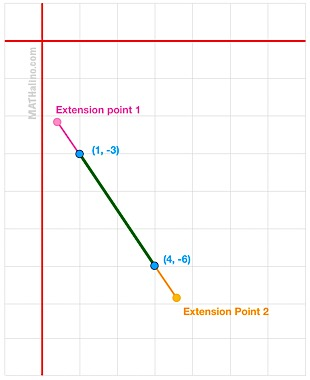Finding the terminal points

5 posts / 0 new
Ralph Lauren Al...Finding the terminal points

The segment joining (1 -3) and (4 -6) is extended a distance equal to one sixth of its own length. find the terminal points.
How to solve this problem. I need the whole SOLUTION. THANKS

Jhun VertThere are two possible extension points as shown. Use the following formulas to find these points:
$x = x_1 + r(x_2 - x_1)$

$y = y_1 + r(y_2 - y_1)$

Note:
(x1, y1) = (1, -3) and
(x2, y2) = (4, -6)For extension point 1, use r = -1/6; and for extension point 2, use r = 7/6.

Anonymous (guest)

How did you find the r=-1/6 and 6/7 then?

Jhun VertConsider the original line (green) to be composed of six parts so that extensions are 1 part each. From (1, -3) to Extension point 1 is -1 part and from (1, -3) to (4, 6) is 6 parts, hence, r = -1/6. Do similarly to Extension Point 2.

Anonymous (guest)

Got it now thank you!

• Mathematics inside the configured delimiters is rendered by MathJax. The default math delimiters are $$...$$ and $...$ for displayed mathematics, and $...$ and $...$ for in-line mathematics.